# Chapter 13 Recursion Copyright 2016 Pearson Inc All

• Slides: 37
Download presentationChapter 13 Recursion Copyright © 2016 Pearson, Inc. All rights reserved.Learning Objectives • Recursive void Functions – Tracing recursive calls – Infinite recursion, overflows • Recursive Functions that Return a Value – Powers function • Thinking Recursively – Recursive design techniques – Binary search Copyright © 2016 Pearson Inc. All rights reserved. 13 -2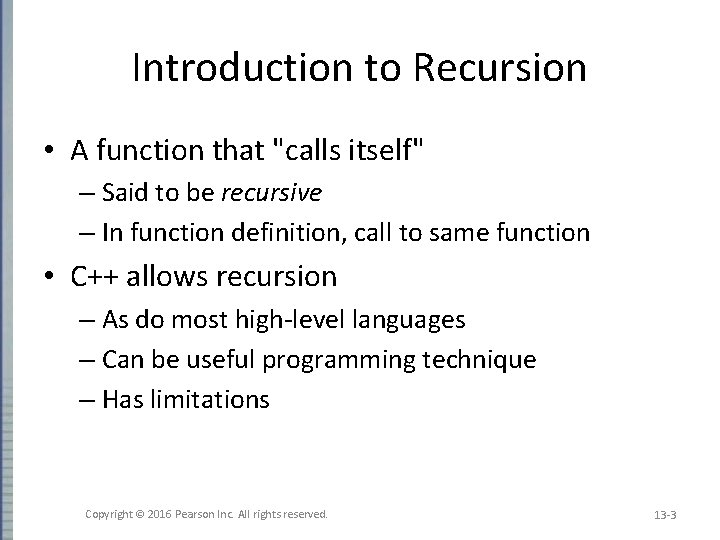Introduction to Recursion • A function that "calls itself" – Said to be recursive – In function definition, call to same function • C++ allows recursion – As do most high-level languages – Can be useful programming technique – Has limitations Copyright © 2016 Pearson Inc. All rights reserved. 13 -3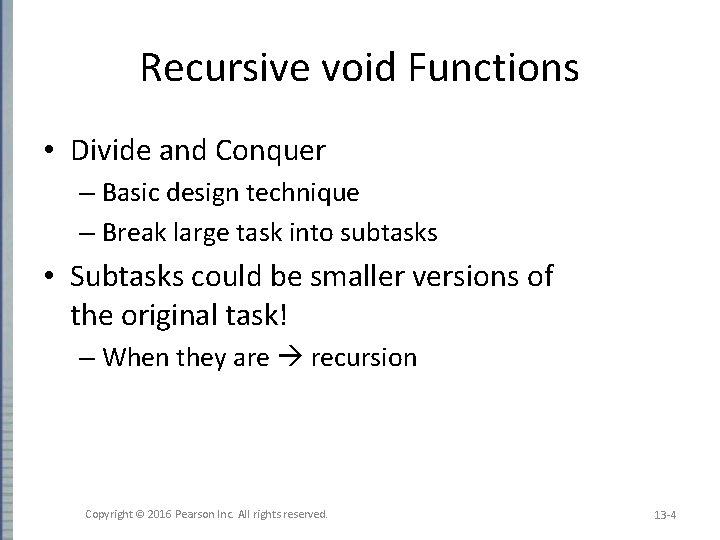Recursive void Functions • Divide and Conquer – Basic design technique – Break large task into subtasks • Subtasks could be smaller versions of the original task! – When they are recursion Copyright © 2016 Pearson Inc. All rights reserved. 13 -4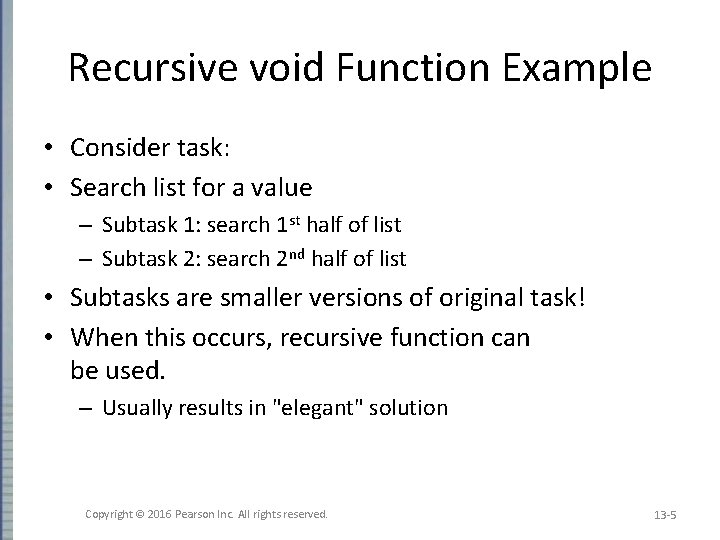Recursive void Function Example • Consider task: • Search list for a value – Subtask 1: search 1 st half of list – Subtask 2: search 2 nd half of list • Subtasks are smaller versions of original task! • When this occurs, recursive function can be used. – Usually results in "elegant" solution Copyright © 2016 Pearson Inc. All rights reserved. 13 -5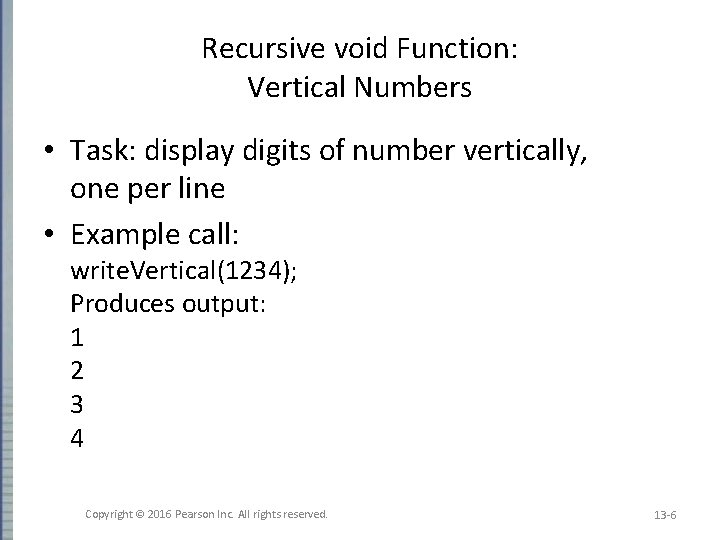Recursive void Function: Vertical Numbers • Task: display digits of number vertically, one per line • Example call: write. Vertical(1234); Produces output: 1 2 3 4 Copyright © 2016 Pearson Inc. All rights reserved. 13 -6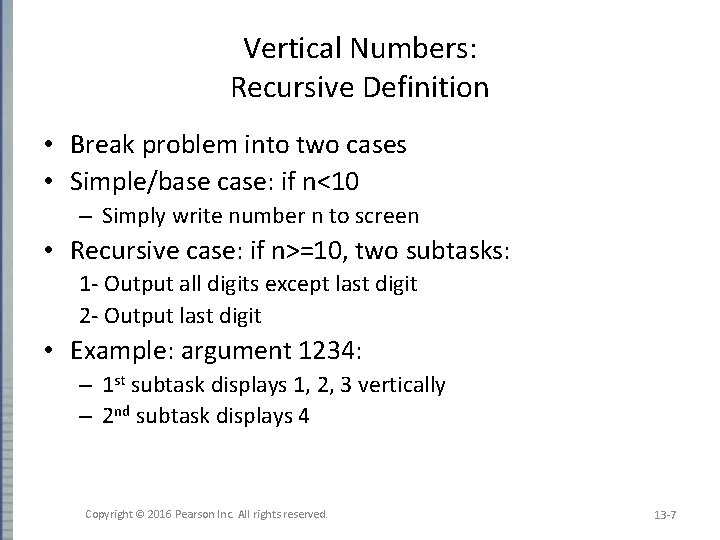Vertical Numbers: Recursive Definition • Break problem into two cases • Simple/base case: if n<10 – Simply write number n to screen • Recursive case: if n>=10, two subtasks: 1 - Output all digits except last digit 2 - Output last digit • Example: argument 1234: – 1 st subtask displays 1, 2, 3 vertically – 2 nd subtask displays 4 Copyright © 2016 Pearson Inc. All rights reserved. 13 -7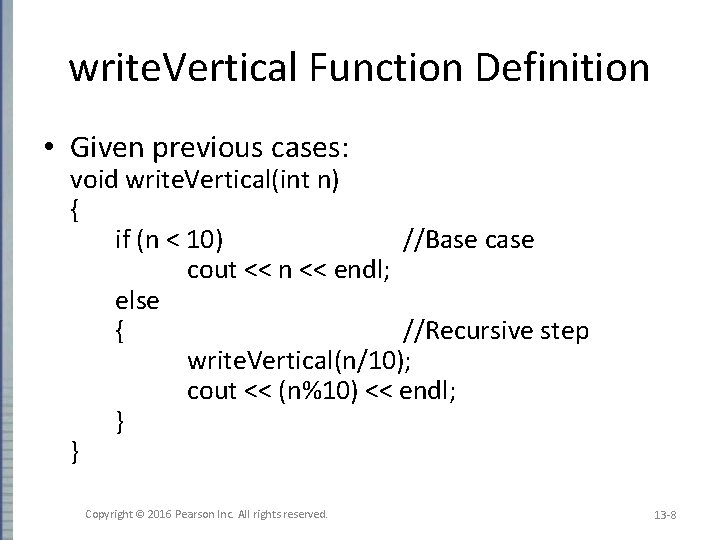write. Vertical Function Definition • Given previous cases: void write. Vertical(int n) { if (n < 10) //Base cout << n << endl; else { //Recursive step write. Vertical(n/10); cout << (n%10) << endl; } } Copyright © 2016 Pearson Inc. All rights reserved. 13 -8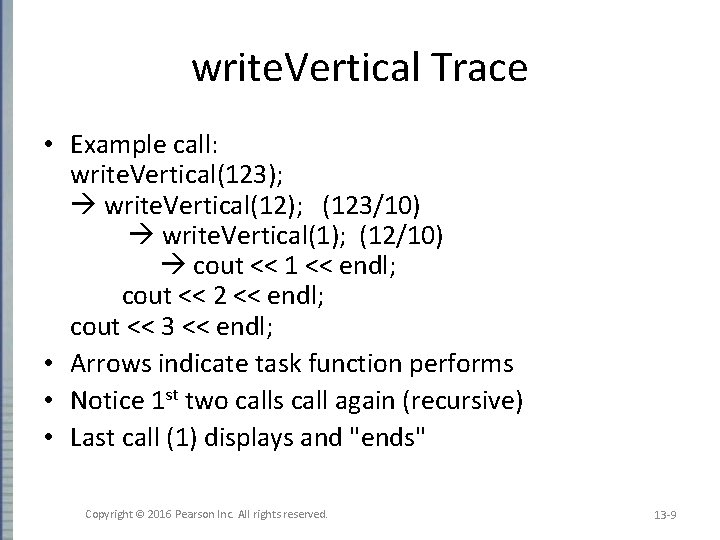write. Vertical Trace • Example call: write. Vertical(123); write. Vertical(12); (123/10) write. Vertical(1); (12/10) cout << 1 << endl; cout << 2 << endl; cout << 3 << endl; • Arrows indicate task function performs • Notice 1 st two calls call again (recursive) • Last call (1) displays and "ends" Copyright © 2016 Pearson Inc. All rights reserved. 13 -9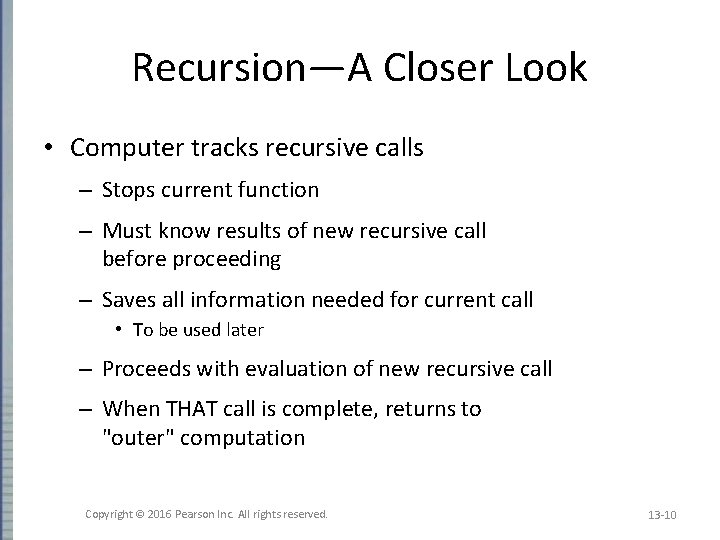Recursion—A Closer Look • Computer tracks recursive calls – Stops current function – Must know results of new recursive call before proceeding – Saves all information needed for current call • To be used later – Proceeds with evaluation of new recursive call – When THAT call is complete, returns to "outer" computation Copyright © 2016 Pearson Inc. All rights reserved. 13 -10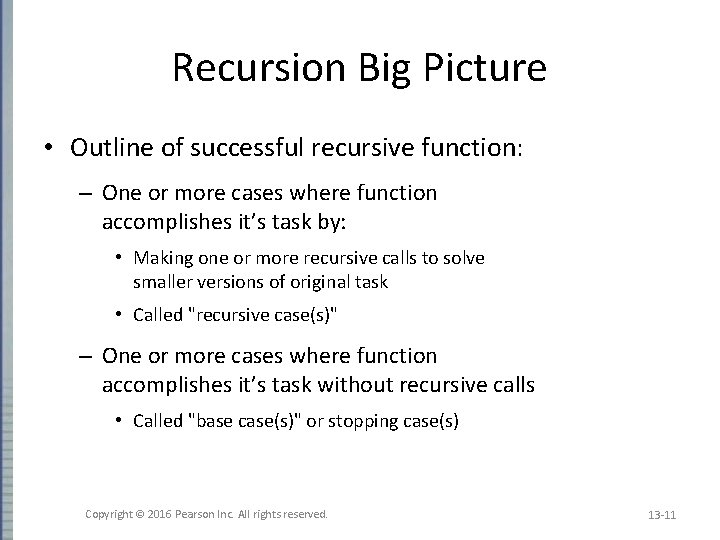Recursion Big Picture • Outline of successful recursive function: – One or more cases where function accomplishes it’s task by: • Making one or more recursive calls to solve smaller versions of original task • Called "recursive case(s)" – One or more cases where function accomplishes it’s task without recursive calls • Called "base case(s)" or stopping case(s) Copyright © 2016 Pearson Inc. All rights reserved. 13 -11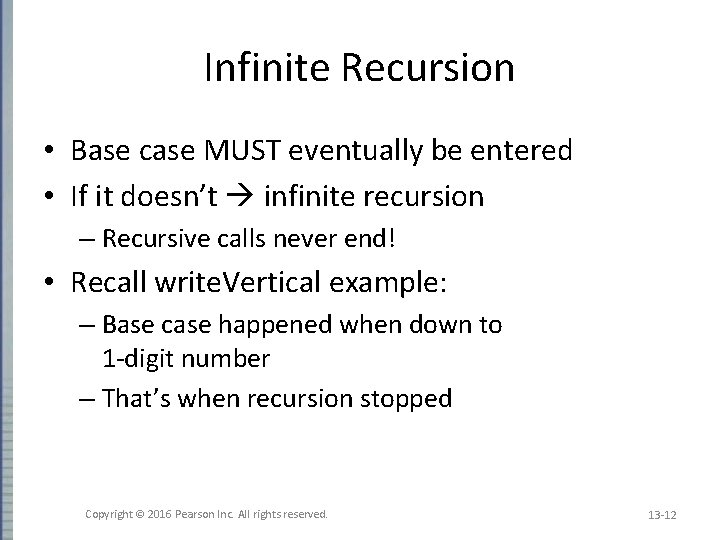Infinite Recursion • Base case MUST eventually be entered • If it doesn’t infinite recursion – Recursive calls never end! • Recall write. Vertical example: – Base case happened when down to 1 -digit number – That’s when recursion stopped Copyright © 2016 Pearson Inc. All rights reserved. 13 -12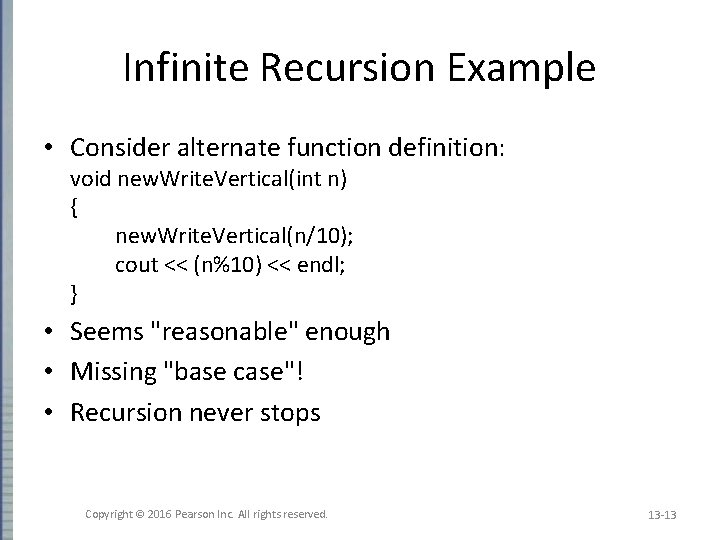Infinite Recursion Example • Consider alternate function definition: void new. Write. Vertical(int n) { new. Write. Vertical(n/10); cout << (n%10) << endl; } • Seems "reasonable" enough • Missing "base case"! • Recursion never stops Copyright © 2016 Pearson Inc. All rights reserved. 13 -13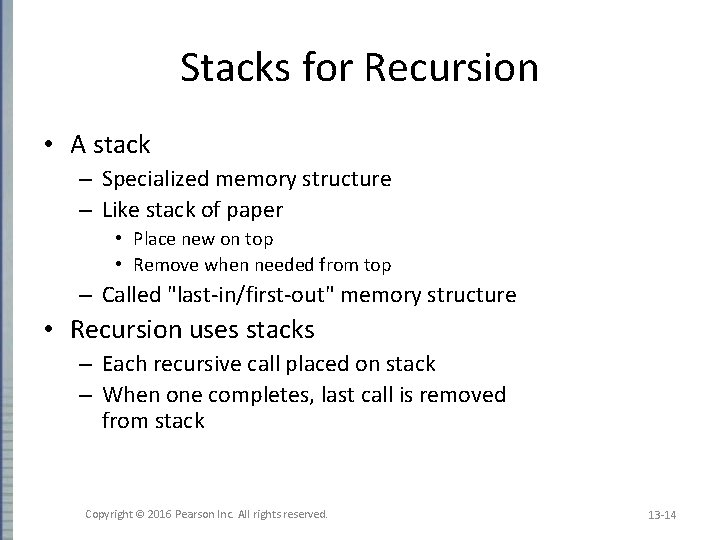Stacks for Recursion • A stack – Specialized memory structure – Like stack of paper • Place new on top • Remove when needed from top – Called "last-in/first-out" memory structure • Recursion uses stacks – Each recursive call placed on stack – When one completes, last call is removed from stack Copyright © 2016 Pearson Inc. All rights reserved. 13 -14Stack Overflow • Size of stack limited – Memory is finite • Long chain of recursive calls continually adds to stack – All are added before base causes removals • If stack attempts to grow beyond limit: – Stack overflow error • Infinite recursion always causes this Copyright © 2016 Pearson Inc. All rights reserved. 13 -15Recursion Versus Iteration • Recursion not always "necessary" • Not even allowed in some languages • Any task accomplished with recursion can also be done without it – Nonrecursive: called iterative, using loops • Recursive: – Runs slower, uses more storage – Elegant solution; less coding Copyright © 2016 Pearson Inc. All rights reserved. 13 -16Recursive Functions that Return a Value • • • Recursion not limited to void functions Can return value of any type Same technique, outline: 1. One+ cases where value returned is computed by recursive calls • Should be "smaller" sub-problems 2. One+ cases where value returned computed without recursive calls • Base case Copyright © 2016 Pearson Inc. All rights reserved. 13 -17Return a Value Recursion Example: Powers • Recall predefined function pow(): result = pow(2. 0, 3. 0); – Returns 2 raised to power 3 (8. 0) – Takes two double arguments – Returns double value • Let’s write recursively – For simple example Copyright © 2016 Pearson Inc. All rights reserved. 13 -18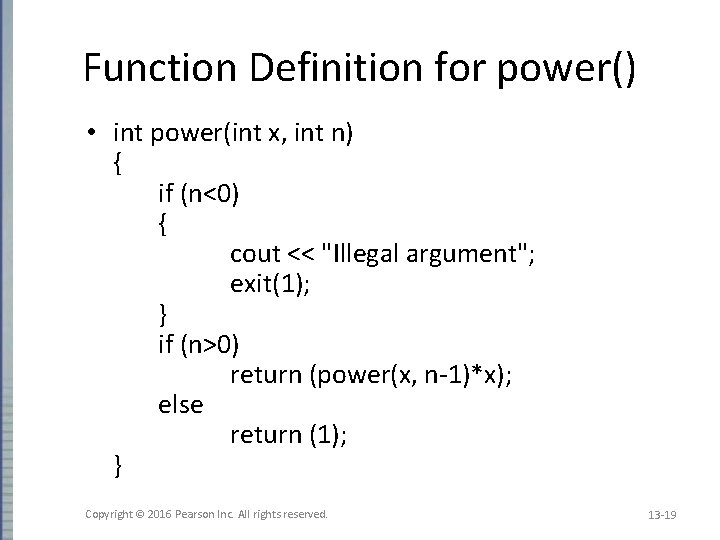Function Definition for power() • int power(int x, int n) { if (n<0) { cout << "Illegal argument"; exit(1); } if (n>0) return (power(x, n-1)*x); else return (1); } Copyright © 2016 Pearson Inc. All rights reserved. 13 -19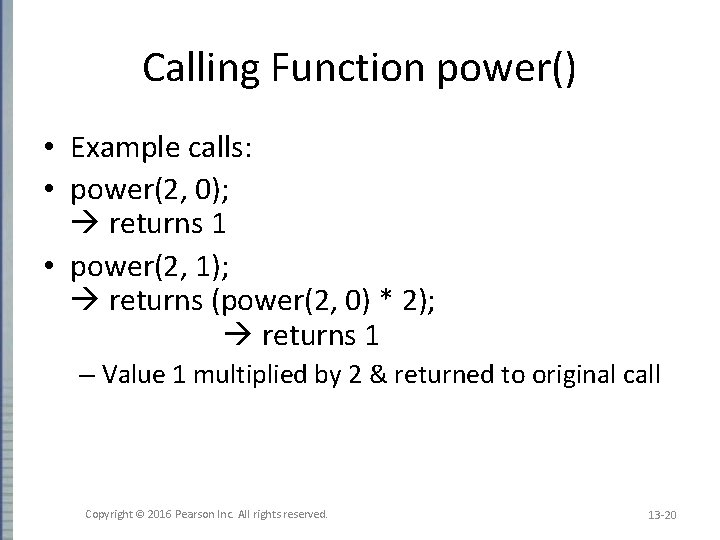Calling Function power() • Example calls: • power(2, 0); returns 1 • power(2, 1); returns (power(2, 0) * 2); returns 1 – Value 1 multiplied by 2 & returned to original call Copyright © 2016 Pearson Inc. All rights reserved. 13 -20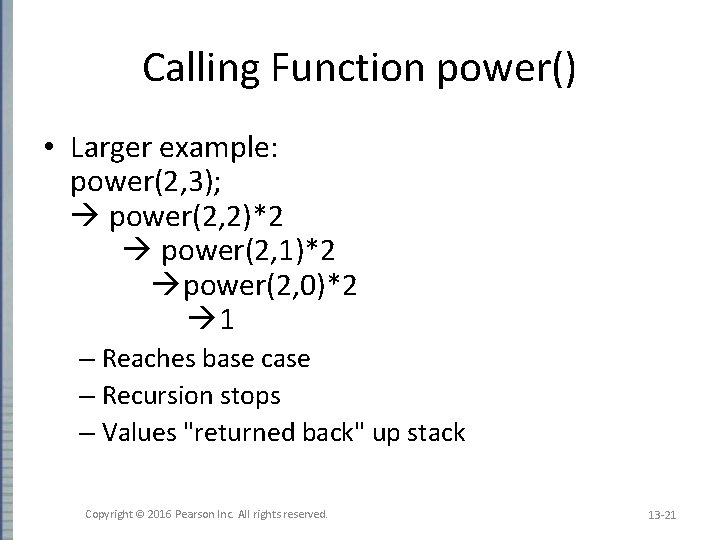Calling Function power() • Larger example: power(2, 3); power(2, 2)*2 power(2, 1)*2 power(2, 0)*2 1 – Reaches base case – Recursion stops – Values "returned back" up stack Copyright © 2016 Pearson Inc. All rights reserved. 13 -21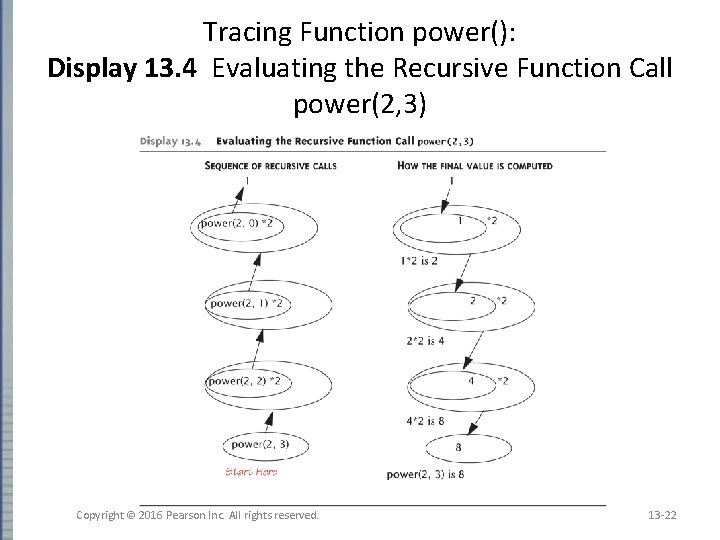Tracing Function power(): Display 13. 4 Evaluating the Recursive Function Call power(2, 3) Copyright © 2016 Pearson Inc. All rights reserved. 13 -22Thinking Recursively • Ignore details – Forget how stack works – Forget the suspended computations – Yes, this is an "abstraction" principle! – And encapsulation principle! • Let computer do "bookkeeping" – Programmer just think "big picture" Copyright © 2016 Pearson Inc. All rights reserved. 13 -23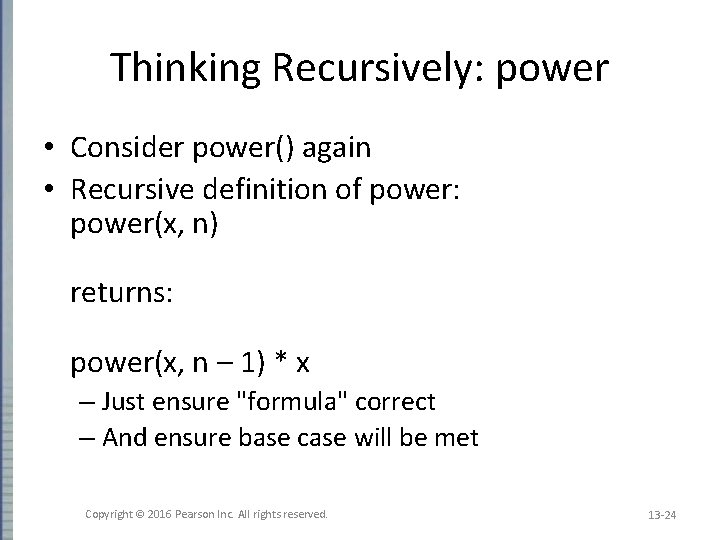Thinking Recursively: power • Consider power() again • Recursive definition of power: power(x, n) returns: power(x, n – 1) * x – Just ensure "formula" correct – And ensure base case will be met Copyright © 2016 Pearson Inc. All rights reserved. 13 -24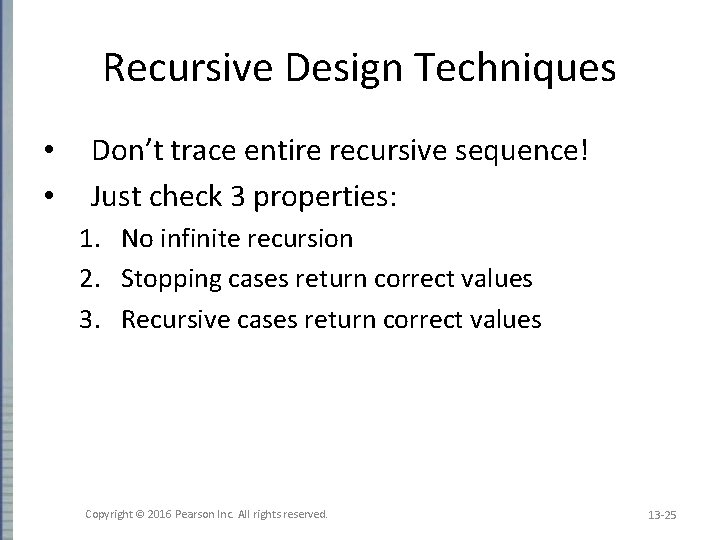Recursive Design Techniques • • Don’t trace entire recursive sequence! Just check 3 properties: 1. No infinite recursion 2. Stopping cases return correct values 3. Recursive cases return correct values Copyright © 2016 Pearson Inc. All rights reserved. 13 -25Recursive Design Check: power() • Check power() against 3 properties: 1. No infinite recursion: • • 2 nd argument decreases by 1 each call Eventually must get to base case of 1 2. Stopping case returns correct value: • • power(x, 0) is base case Returns 1, which is correct for x 0 3. Recursive calls correct: • • For n>1, power(x, n) returns power(x, n-1)*x Plug in values correct Copyright © 2016 Pearson Inc. All rights reserved. 13 -26Tail recursion • A function that is tail recursive if it has the property that no further computation occurs after the recursive call; the function immediately returns. • Tail recursive functions can easily be converted to a more efficient iterative solution – May be done automatically by your compiler Copyright © 2016 Pearson Inc. All rights reserved. 13 -27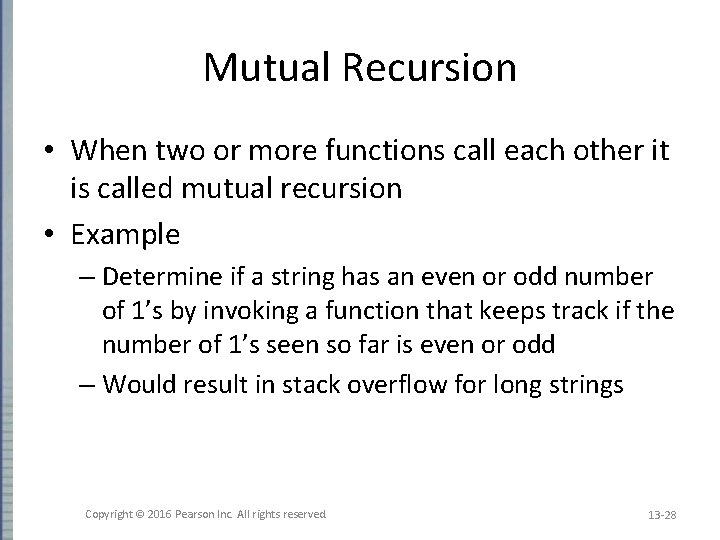Mutual Recursion • When two or more functions call each other it is called mutual recursion • Example – Determine if a string has an even or odd number of 1’s by invoking a function that keeps track if the number of 1’s seen so far is even or odd – Would result in stack overflow for long strings Copyright © 2016 Pearson Inc. All rights reserved. 13 -28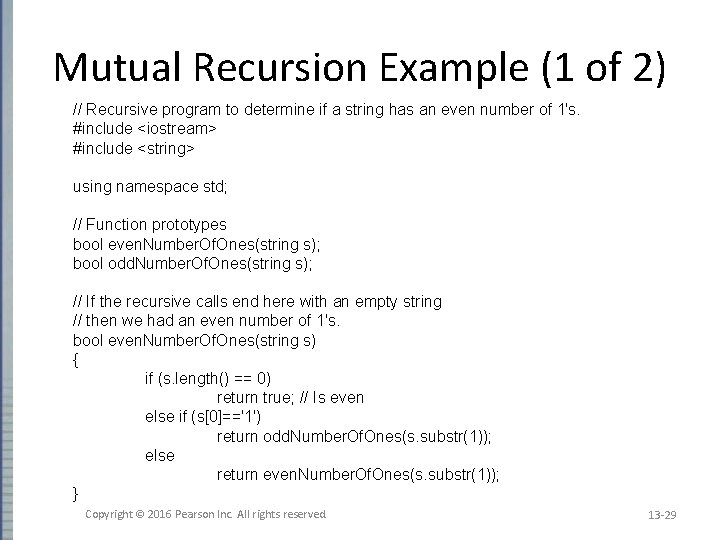Mutual Recursion Example (1 of 2) // Recursive program to determine if a string has an even number of 1's. #include <iostream> #include <string> using namespace std; // Function prototypes bool even. Number. Of. Ones(string s); bool odd. Number. Of. Ones(string s); // If the recursive calls end here with an empty string // then we had an even number of 1's. bool even. Number. Of. Ones(string s) { if (s. length() == 0) return true; // Is even else if (s=='1') return odd. Number. Of. Ones(s. substr(1)); else return even. Number. Of. Ones(s. substr(1)); } Copyright © 2016 Pearson Inc. All rights reserved. 13 -29Mutual Recursion Example (2 of 2) // if the recursive calls end up here with an empty string // then we had an odd number of 1's. bool odd. Number. Of. Ones(string s) { if (s. length() == 0) return false; // Not even else if (s=='1') return even. Number. Of. Ones(s. substr(1)); else return odd. Number. Of. Ones(s. substr(1)); } int main() { string s = "10011"; if (even. Number. Of. Ones(s)) cout << "Even number of ones. " << endl; else cout << "Odd number of ones. " << endl; return 0; } Copyright © 2016 Pearson Inc. All rights reserved. 13 -30Binary Search • Recursive function to search array – Determines IF item is in list, and if so: – Where in list it is • Assumes array is sorted • Breaks list in half – Determines if item in 1 st or 2 nd half – Then searches again just that half • Recursively (of course)! Copyright © 2016 Pearson Inc. All rights reserved. 13 -31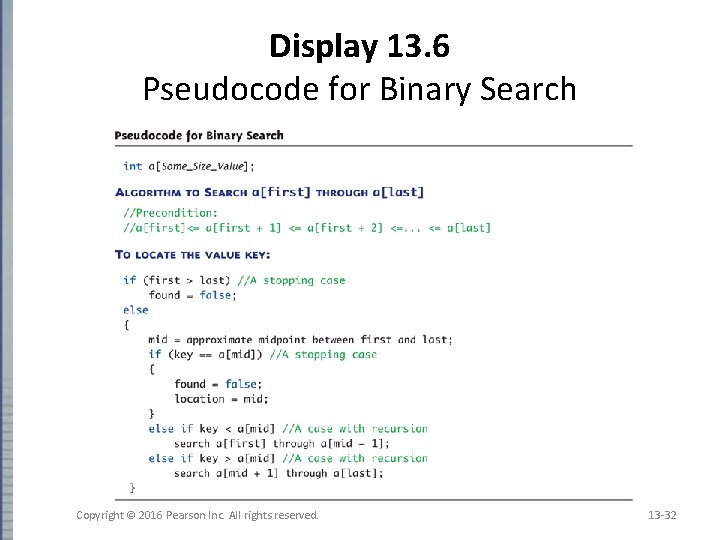Display 13. 6 Pseudocode for Binary Search Copyright © 2016 Pearson Inc. All rights reserved. 13 -32Checking the Recursion • Check binary search against criteria: 1. No infinite recursion: • • Each call increases first or decreases last Eventually first will be greater than last 2. Stopping cases perform correct action: • • If first > last no elements between them, so key can’t be there! IF key == a[mid] correctly found! 3. Recursive calls perform correct action • • If key < a[mid] key in 1 st half – correct call If key > a[mid] key in 2 nd half – correct call Copyright © 2016 Pearson Inc. All rights reserved. 13 -33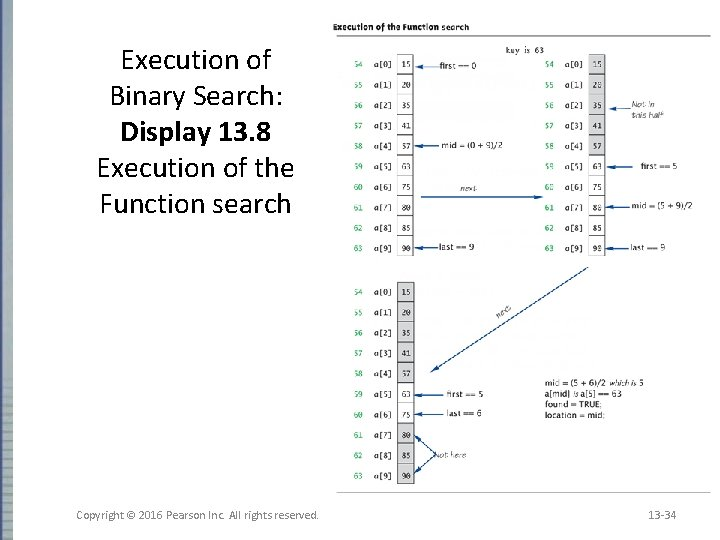Execution of Binary Search: Display 13. 8 Execution of the Function search Copyright © 2016 Pearson Inc. All rights reserved. 13 -34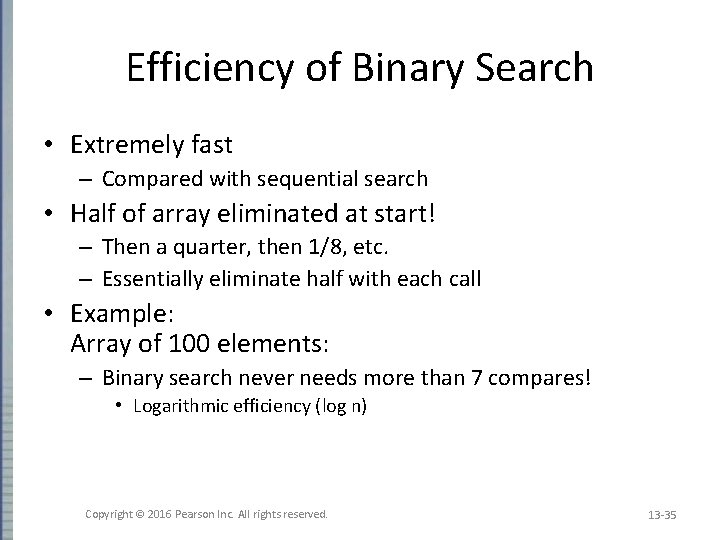Efficiency of Binary Search • Extremely fast – Compared with sequential search • Half of array eliminated at start! – Then a quarter, then 1/8, etc. – Essentially eliminate half with each call • Example: Array of 100 elements: – Binary search never needs more than 7 compares! • Logarithmic efficiency (log n) Copyright © 2016 Pearson Inc. All rights reserved. 13 -35Recursive Solutions • Notice binary search algorithm actually solves "more general" problem – Original goal: design function to search an entire array – Our function: allows search of any interval of array • By specifying bounds first and last • Very common when designing recursive functions Copyright © 2016 Pearson Inc. All rights reserved. 13 -36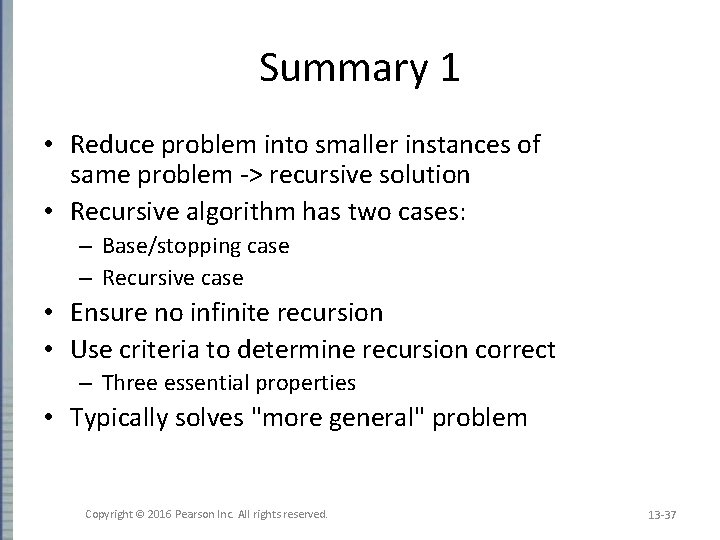Summary 1 • Reduce problem into smaller instances of same problem -> recursive solution • Recursive algorithm has two cases: – Base/stopping case – Recursive case • Ensure no infinite recursion • Use criteria to determine recursion correct – Three essential properties • Typically solves "more general" problem Copyright © 2016 Pearson Inc. All rights reserved. 13 -37